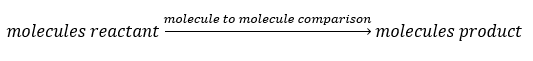# Problem: Consider the following reaction:4 NH3 (g) + 5 O2 (g) → 4 NO (g) + 6 H2O (g)If a container were to have 10 molecules of O 2 and 10 molecules of NH 3 initially, how many total molecules (reactants plus products) would be present in the container after this reaction goes to completion?

###### FREE Expert Solution

From the balance reaction equation, we can do a molecule to molecule comparison to determine how many molecules of products are formed. The limiting reactant would be the reactant that produces smaller number of products.Balanced reaction: 4 NH3(g) + 5 O2(g) → 4 NO(g) + 6 H2O(g)

We will use NO to determine the limiting reactant:

92% (388 ratings)###### Problem Details

Consider the following reaction:

4 NH3 (g) + 5 O2 (g) → 4 NO (g) + 6 H2O (g)

If a container were to have 10 molecules of O 2 and 10 molecules of NH 3 initially, how many total molecules (reactants plus products) would be present in the container after this reaction goes to completion?